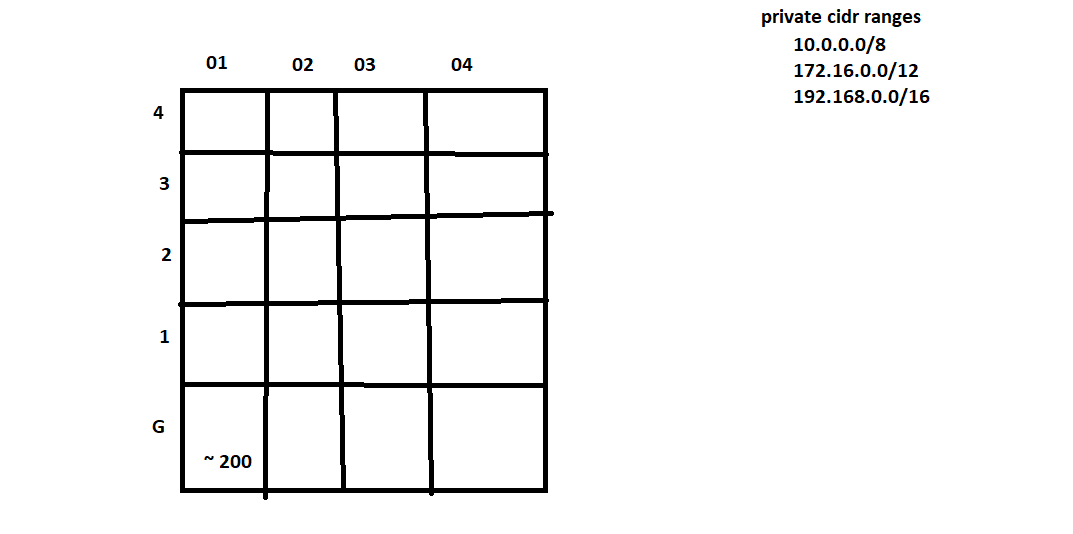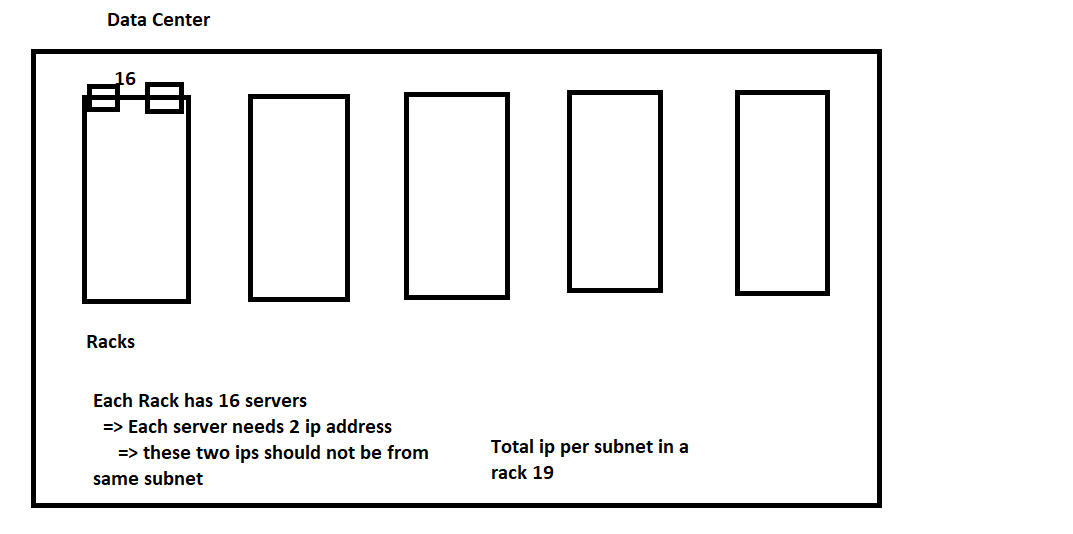## Subnet

• sub-network
• Network break into multiple subnets

## Subnetting

• Dividing Larger network into multiple smaller network is subnetting
• Conventions:
• Subnets network id will contain network’s network id
``````Network: 192.168.0.0/16 => 192.168 (Nid) => 2^16-2

Possible Subnet : 192.168.0.0/24 => 192.168.0 (Nid) => 2^8-2

Possible Subnet: 192.168.0.0/16 => 192.168(Nid)

Impossible Subnet: 192.168.0.0/8 => 192

Impossible Subnet : 192.167.0.0/24 =>

``````

### Design a network with 200 devices per subnet (private network)``````2 power x ~= 200
what can be value of x
x = 8

``````
• Here x stands for host sizes.
• But when we write cidr we need to consider nid size
``````host id size = 8
network id size = 32 -8 = 24

``````
• when you design subnets your cidr range will be x.y.z.0/24 and network can be x.y.0.0/16
• If we consider private cidr range as 10.10.0.0/16
``````G-1 => 10.10.0.0/24
G-2 => 10.10.1.0/24
..
..
404 => 10.10.19.0/24
``````

### Design private network for the below image## How to enable communications between two networks?

``````ipconfig

ip                  192.168.0.11
sm                  255.255.255.0
default gateway     192.168.0.1

``````
• There is a device called as router, which can send packets from one network to another
• At our homes we are able to google.
``````tracert google.com
``````

### Router

• Router forwards packet from one network to another
• Router has something called at route table.
• This route table will consist of all different networks to which packets can be forwarded.

### NAT